Latest Banking jobs   »

# Quantitative Aptitude Quiz For LIC AAO Mains 2023- 17th March

Directions (1-5): Study the following graphs carefully and answer the questions given below:
Graph given below shows the monthly expenditure by 6 persons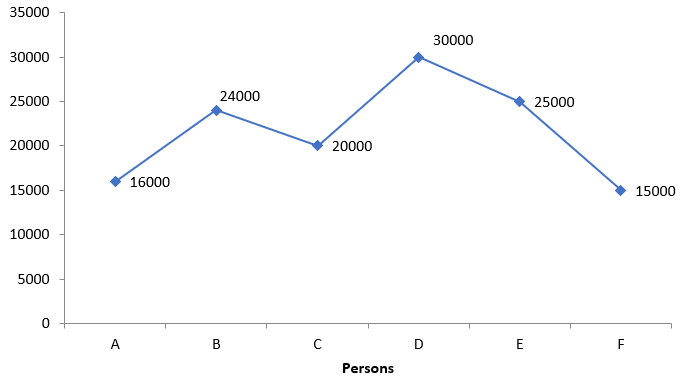Given below is the pie chart for percentage breakup of monthly Income of person B.
Note- Income = Expenditure + Saving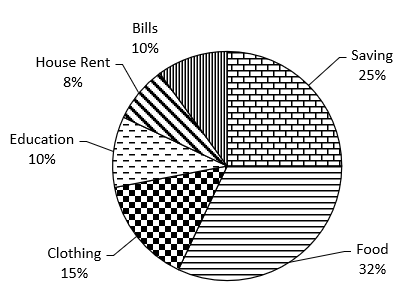Q1. If saving of C is equal to the sum of expenditure of E on clothing and bill together and expenditure of E on Bill is 25% less than expenditure of B on bill then what is the sum of savings of C and B together, given that the ratio of expenditure of E on clothing and Bill is 5 : 3.
(a) 13250
(b) 14400
(c) 15550
(d) 12560
(e) None of these
Q2. If expenditure of A and C on clothing is 25% and 15% respectively of their expenditure and saving of A is 25% more than expenditure of A on clothing, then sum of saving of A and expenditure of C on clothing together is what percent of expenditure of B on clothing?
(a) 155%
(b) 180.5%
(c) 160.75%
(d) 166.67%
(e) 200%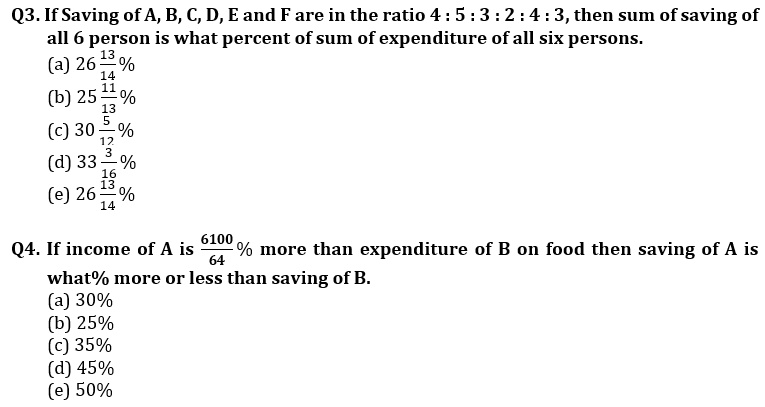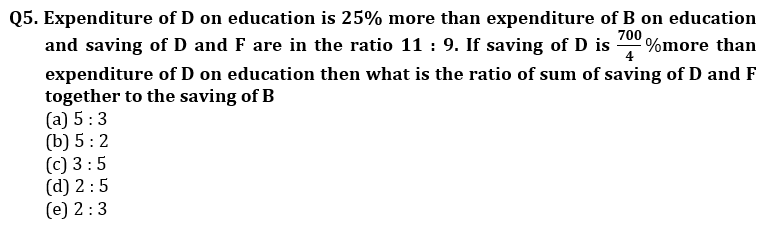Directions (6-10): In each of these questions, two equations (I) and (II) are given. You have to solve both the equations and give answer
(a) if x>y
(b) if x≥y
(c) if x<y
(d) if x ≤y
(e) if x = y or no relation can be established between x and y.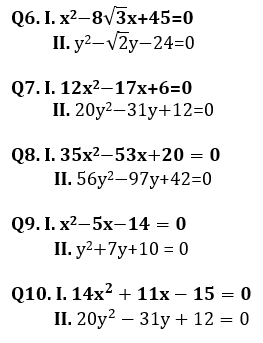Directions (11-15): Study the table carefully to answer the questions that follow
Table below shows the runs scored, Number of matches played, average of runs per innings, total number of 4’ and 6’s hit and ratio of number of fours and sixes hit by five batsmen in women’s world cup (WWC) 2017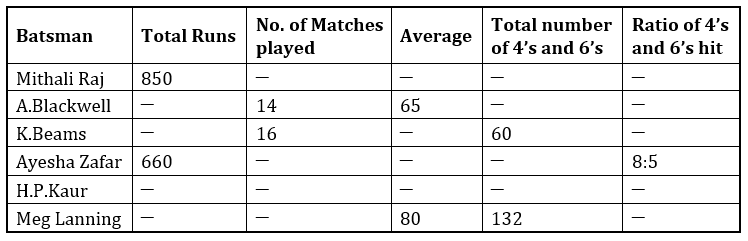Note – (No batsman, remained not out in any innings)
Few values are missing in table, a candidate is expected to calculate the missing values if it is required to answer the given questions.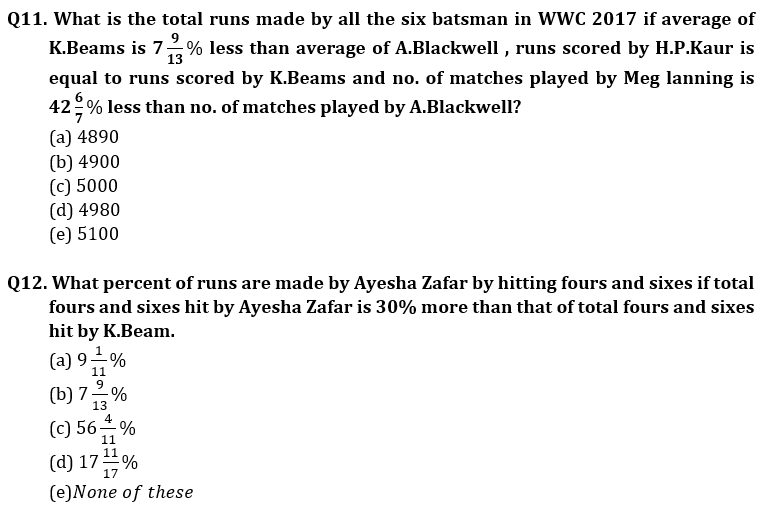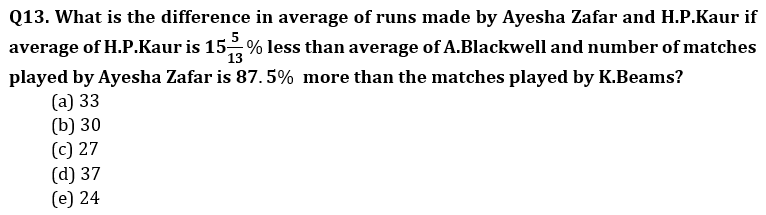Q14. What is the difference between the number of fours hit by Ayesha Zafar to the number of fours hit by Meg Lanning if total four and sixes hit by Ayesha Zafar is 30% more than four and sixes hit by K.Beam and ratio of number of fours to sixes hit by Meg Lanning is 7:4.
(a) 48
(b) 52
(c) 28
(d) 32
(e) 36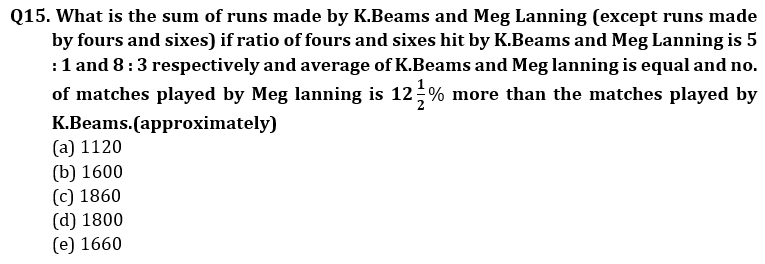Solutions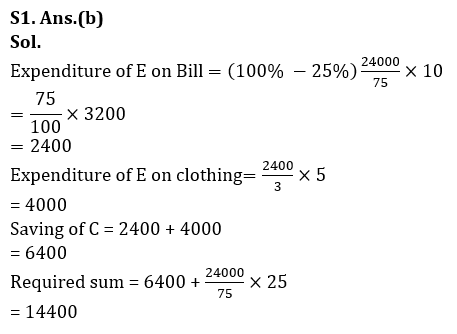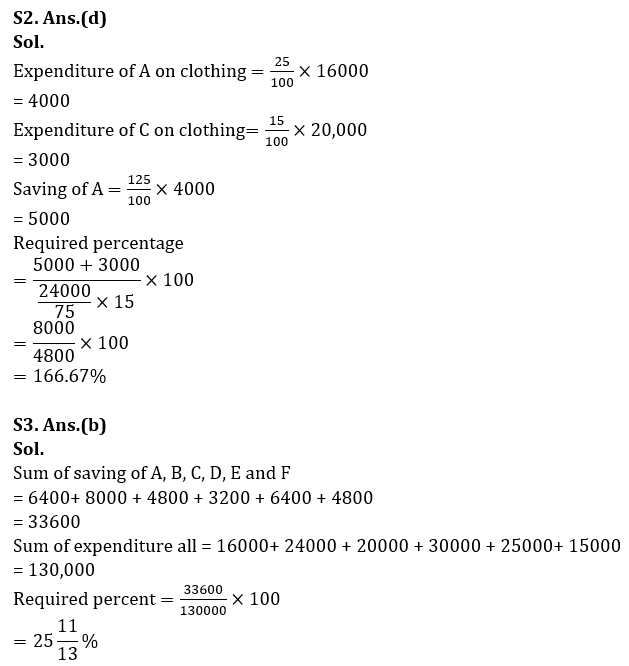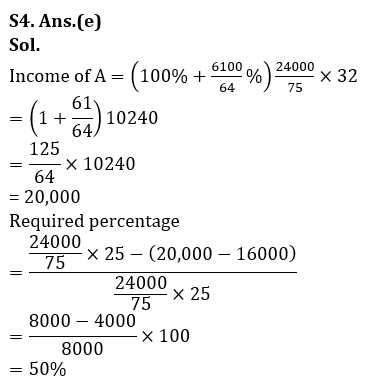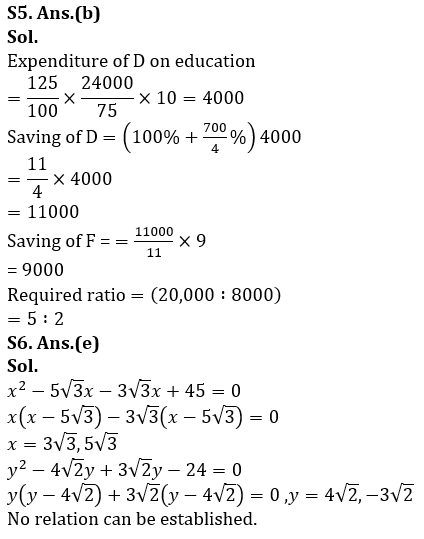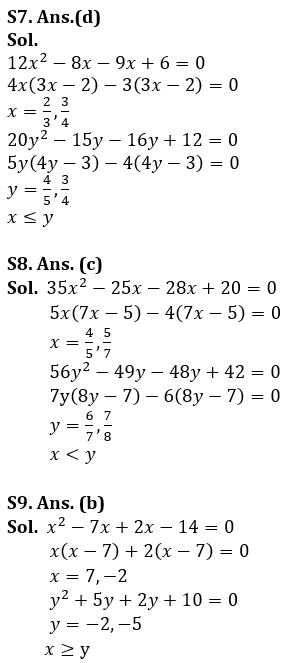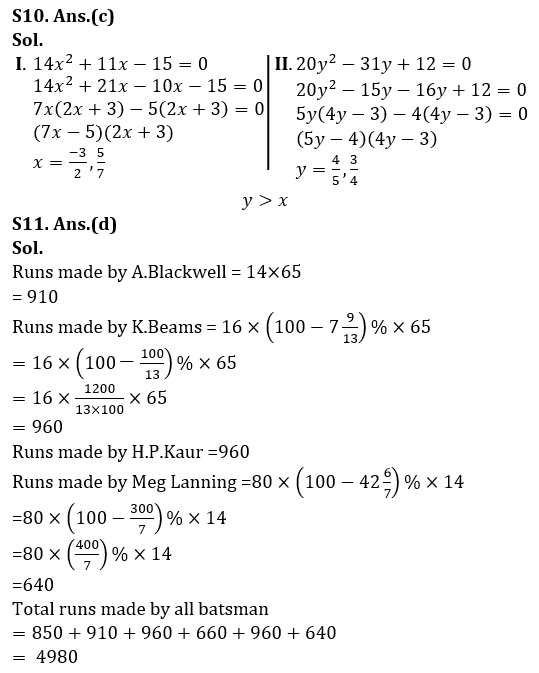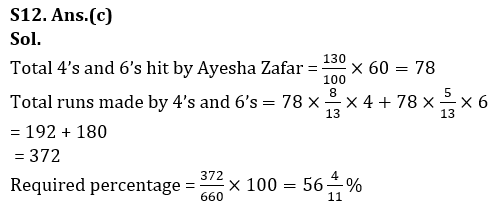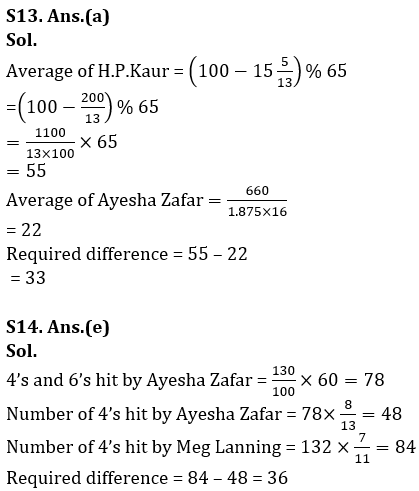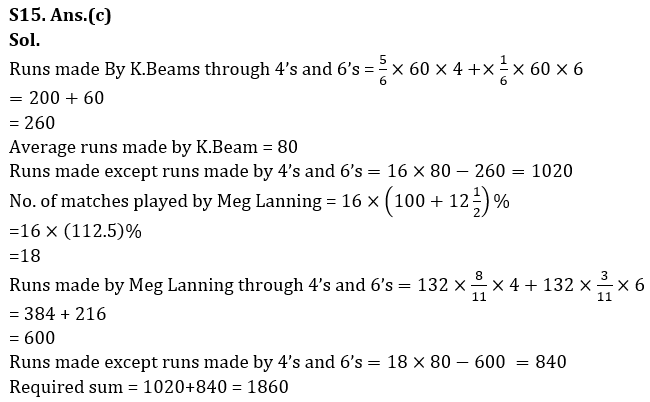## FAQs

### When will the LIC AAO Mains exam be held?

LIC AAO Mains exam will be held on 18 March

#### Congratulations!Union Budget 2023-24: Free PDF